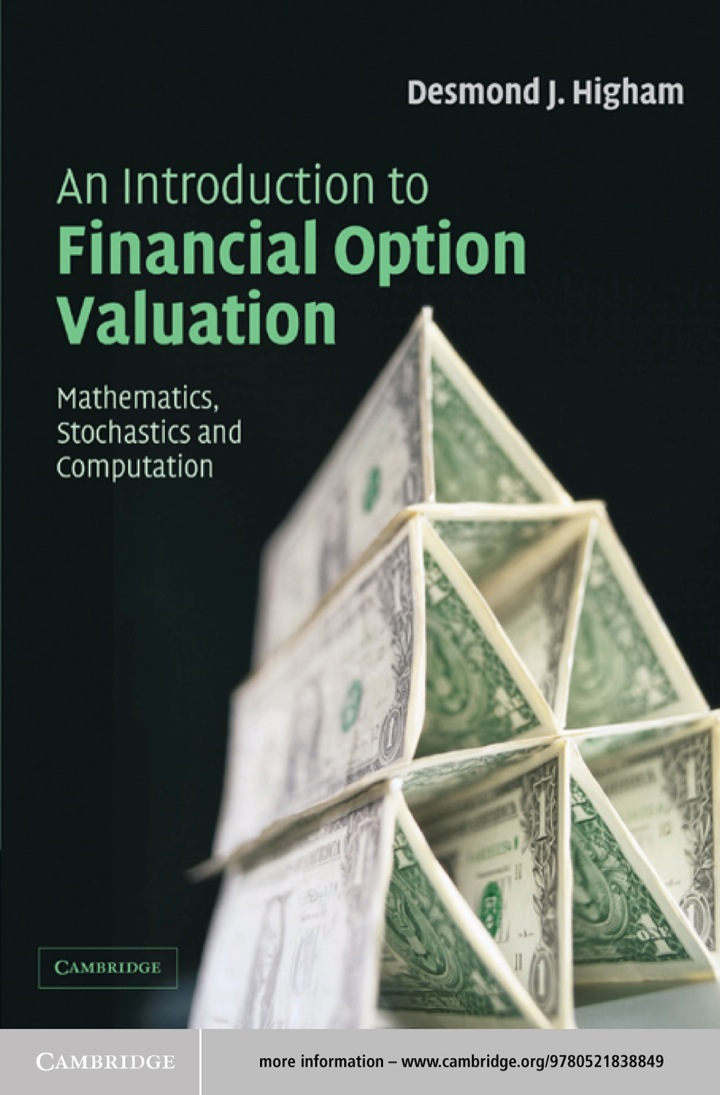# An Introduction to Financial Option Valuation

## An Introduction to Financial Option Valuation

By Desmond J. Higham

### Rent or Buy eTextbook

Expires on Apr 15th, 2022
\$49

Publisher List Price: \$0.00

This is a lively textbook providing a solid introduction to financial option valuation for undergraduate students armed with a working knowledge of a first year calculus. Written in a series of short chapters, its self-contained treatment gives equal weight to applied mathematics, stochastics and computational algorithms. No prior background in probability, statistics or numerical analysis is required. Detailed derivations of both the basic asset price model and the Black–Scholes equation are provided along with a presentation of appropriate computational techniques including binomial, finite differences and in particular, variance reduction techniques for the Monte Carlo method. Each chapter comes complete with accompanying stand-alone MATLAB code listing to illustrate a key idea. Furthermore, the author has made heavy use of figures and examples, and has included computations based on real stock market data.

Subject: Mathematics & Statistics -> Mathematics -> Mathematics GeneralMathematics, Stochastics and Computation
1st edition
Publisher: Cambridge University Press 4/15/04
Imprint: Cambridge University Press
Language: English

ISBN 10: 1139637185
ISBN 13: 9781139637183
Print ISBN: 9780521547574

Live Chats